# Formal language

in mathematical linguistics

An arbitrary set of chains (that is, words, cf. Word) over some (finite or infinite) alphabet(sometimes also called a dictionary), that is, a set of expressions of the form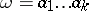, where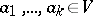; the number, usually denoted by, is the length of the chain. One also considers the empty chain, denoted by; one sets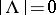. One often speaks of a language over the alphabet, omitting the word "formal" . In mathematical linguistics and the theory of automata (cf. Automata, theory of) one considers various effective ways of specifying a formal language, principally by means of formal grammars (cf. Grammar, formal) and automata of various types, which can, in the majority of cases, be described as modifications of non-deterministic Turing machines (cf. Turing machine), often multi-tape, with some restrictions on the ways the machine works on each tape.

## Operations on formal languages.

In addition to the usual set-theoretic operations on formal languages, one carries out: multiplication (or direct multiplication, or concatenation):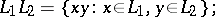left division:right division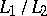is defined by analogy with left division; iteration:where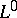denotesand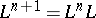(in particular, the set of all chains inis); truncated iteration: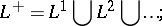and substitution: Ifis a language over the finite alphabet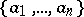andare arbitrary languages, then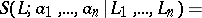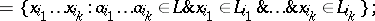if each of the languages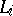,, consists of one chain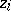, a substitution is called a homomorphism; if all theare non-empty, one speaks of an unabbreviated homomorphism. If the languageconsists of one chain, then instead of,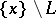, etc., one writes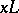,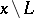, etc., respectively.

A variety of languages is an ordered pair(or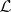, if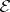is taken for granted), whereis an infinite alphabet andis a class of languages such that: 1) for every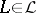there is a finite alphabet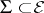such that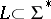; 2)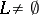for some; and 3)is closed relative to union, multiplication, intersection with regular sets, truncated iteration, unabbreviated homomorphisms, and inverses of arbitrary homomorphisms. A variety that is closed relative to arbitrary homomorphisms is called complete.